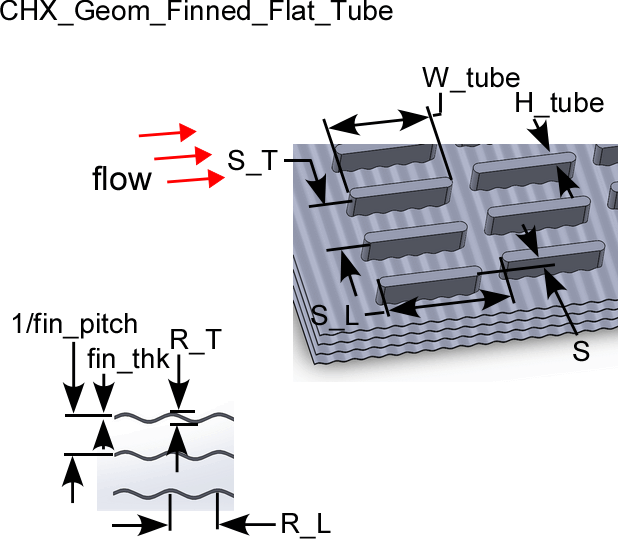Contents

Finned flat tubes geometryThe procedure:

CHX_Geom_Finned_Flat_Tube(TypeHX\$: H_tube, W_tube, S_T, S_L, S, fin_pitch, fin_thk, R_T, R_L, D_h, sigma, alpha, A_fin\A)

Provides the geometric data associated with a finned flat tube compact heat exchanger surface.  These data are from Kays and London (1994).

Inputs:

The only input is the string identifying the geometry TypeHX\$

FF-9.68-0.87 : 'ff_tubes_s968_087'

FF-9.1-0.737-S : 'ff_tubes_s91_0737_s'

FF-9.68-0.87-R : 'ff_tubes_s968_087_r'

FF-9.29-0.737-SR : 'ff_tubes_s929_0737_sr'

FF-11.32-0.737-SR : 'ff_tubes_s1132_0737_sr'

Outputs:

H_tube = tube dimension as viewed from flow direction (m or ft)

W_tube = tube dimension as viewed perpendicular to flow direction (m or ft)

S_T = transverse tube spacing (m or ft)

S_L = longitudinal tube spacing (m or ft)

S = stagger distance (m or ft) = 0 for unstaggered geometries

fin_pitch = # fins per length (fins/m or fins/ft)

fin_thk = fin thickness (m or ft)

R_T = fin ripple amplitude in transverse direction (m or ft) = 0 for unrippled fins

R_L = fin ripple amplitude in longitudinal direction (m or ft) = 0 for unrippled fins

D_h = hydraulic diameter defined as 4 x minimum flow area x length/heat transfer area (m or ft)

sigma = minimum flow area/frontal area

alpha = heat transfer area/volume (m^2/m^3 or ft^2/ft^3)

A_fin\A = heat transfer area on fins/heat transfer area

Example

\$UnitSystem SI Mass J K Pa

TypeHX\$='ff_tubes_s968_087_R'

Call chx_geom_finned_flat_tube(TypeHX\$: H_tube, W_tube, S_T, S_L, S, fin_pitch, fin_thk, R_T, R_L, D_h, sigma, alpha, A_fin\A)

{Solution is:

H_tube = 0.00305 [m]

W_tube = 0.0221 [m]

S_T = 0.011 [m]

S_L = 0.0269 [m]

S = 0 [m]

fin_pitch = 381 [1/m]

fin_thk = 0.00010 [m]

R_T = 0.000635 [m]

R_L = 0.00635 [m]

D_h = 0.003597 [m]

sigma = 0.697

alpha = 751 [m^2/m^3]

A_fin\A = 0.795}

Related procedures include:

Nondimensional Functions

Coefficient of Heat Transfer